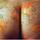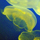## Social Question# Can you figure out how to do this renumbering?

Asked by LostInParadise (28133) June 25th, 2010

This problem gets its inspiration from something that came up at work.

A company creates batch and sequence numbers for each product. To keep things simple, suppose that the batch number is four digits and the sequence number is one digit. The obvious way to get from one batch number/sequence number is to treat the pair as a single five digit number and add one. For example to get from 0743/9 is to form 7439+1= 7440, giving 0744/0. This is what the programmer in fact did, but there is one small hitch. The sequence numbers go from 1 to 9, no zeroes. So in the above example, the next assignment would be 0744/1.

The programming change is not hard, just add another 1 if you get a zero, but what about the numbers that have already been assigned? Can you think of a way of converting 0072/1 under the original numbering to what it should be?

Observing members: 0Composing members: 0if statements

wundayatta (58635)“Great Answer” (0) Flag as…If I follow you correctly, numbering scheme #1 should be like the left column, and should map towards numbering scheme #2 as in the second column.

0000/0 => 1111/1
...
0000/8 => 1111/9
0000/9 => 1112/1
0001/0 => 1112/2
0001/1 => 1112/3
....
0001/7 => 1112/9
0001/8 => 1113/1
...

This could be accomplished by treating the numbers as 5-digit integers, and adding 11111 to the original number, plus another 1 for each time it has passed around 9, or, in pseudocode:

newnum=orignum+11111+floor(orignum/9)

For example

0000/9 => 00009+11111+floor(00009/9)=11121 => 1112/1
0001/8 => 00018+11111+floor(00018/9)=11131 => 1113/1

0072/1 => 00721+11111+floor(00721/9)=11912 => 1191/2

Correct?

nisse (1986)“Great Answer” (1) Flag as…I should have explained the problem better. For the batch number, 0000 is not permitted, but otherwise 0 can be used as usual. 0001 and 0010 are legitimate batch numbers. However, your solution is correct and could be easily modified for how I should have stated the problem.

LostInParadise (28133)“Great Answer” (0) Flag as…Ah, I see that in the examples now. Exchange the solution with
newnum=orignum+1+floor(orignum/9)

0000/9 => 00009+1+floor(00009/9)=00011 => 0001/1
0001/8 => 00018+1+floor(00018/9)=00021 => 0002/1

0072/1 => 00721+1+floor(00721/9)=00802 => *0080/2

Anyhoo, i thought it was a fun problem, albeit apparently not a crowdpleaser :)

nisse (1986)“Great Answer” (0) Flag as…